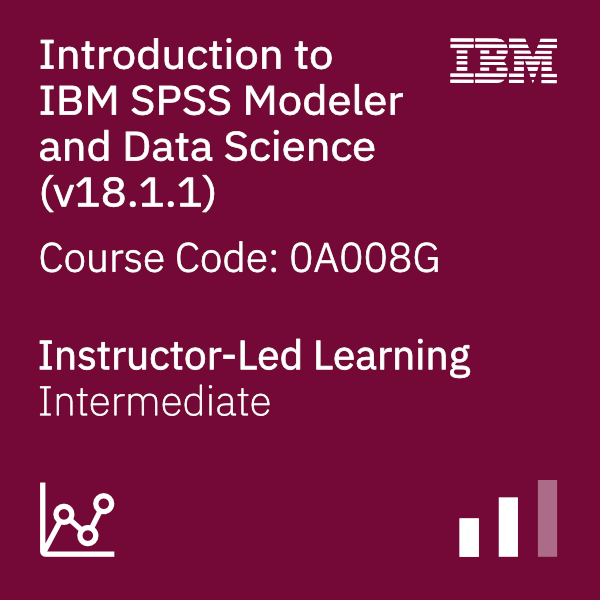Overview

This course provides the fundamentals of using IBM SPSS Modeler and introduces the participant to data science. The principles and practice of data science are illustrated using the CRISP-DM methodology. The course provides training in the basics of how to import, explore, and prepare data with IBM SPSS Modeler v18.1.1, and introduces the student to modeling.

Audience

• Data scientists
• Clients who are new to IBM SPSS Modeler or want to find out more about using it

Prerequisites

• It is recommended that you have an understanding of your business data

Objective

• Introduction to data science
• Introduction to IBM SPSS Modeler
• Introduction to data science using IBM SPSS Modeler
• Collecting initial data
• Understanding the data
• Setting the of analysis
• Integrating data
• Deriving and reclassifying fields
• Identifying relationships
• Introduction to modeling

Show details

Course Outline

1. Introduction to data science
• List two applications of data science
• Explain the stages in the CRISP-DM methodology
• Describe the skills needed for data science
2. Introduction to IBM SPSS Modeler
• Describe IBM SPSS Modeler's user-interface
• Work with nodes and streams
• Generate nodes from output
• Use SuperNodes
• Execute streams
• Open and save streams
• Use Help
3. Introduction to data science using IBM SPSS Modeler
• Explain the basic framework of a data-science project
• Build a model
• Deploy a model
4. Collecting initial data
• Explain the concepts "data structure", "of analysis", "field storage" and "field measurement level"
• Import Microsoft Excel files
• Import IBM SPSS Statistics files
• Import text files
• Import from databases
• Export data to various formats
5. Understanding the data
• Audit the data
• Check for invalid values
• Take action for invalid values
• Define blanks
6. Setting the of analysis
• Remove duplicate records
• Aggregate records
• Expand a categorical field into a series of flag fields
• Transpose data
7. Integrating data
• Append records from multiple datasets
• Merge fields from multiple datasets
• Sample records
8. Deriving and reclassifying fields
• Use the Control Language for Expression Manipulation (CLEM)
• Derive new fields
• Reclassify field values
9. Identifying relationships
• Examine the relationship between two categorical fields
• Examine the relationship between a categorical field and a continuous field
• Examine the relationship between two continuous fields
10. Introduction to modeling
• List three types of models
• Use a supervised model
• Use a segmentation model